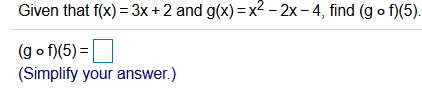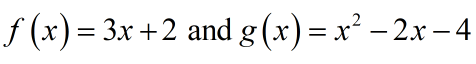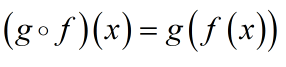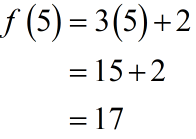# Given that f(x) = 3x+2 and g(x) =x2 - 2x - 4, find (g o f)(5).(g o f)(5) =(Simplify your answer.)

Question
1 viewshelp_outlineImage TranscriptioncloseGiven that f(x) = 3x+2 and g(x) =x2 - 2x - 4, find (g o f)(5). (g o f)(5) = (Simplify your answer.) fullscreen
check_circle

Step 1

Consider the given functions as below;Known fact;Calculat the value of f at the point x=5 as follows....

### Want to see the full answer?

See Solution

#### Want to see this answer and more?

Solutions are written by subject experts who are available 24/7. Questions are typically answered within 1 hour.*

See Solution
*Response times may vary by subject and question.
Tagged in

### Algebra